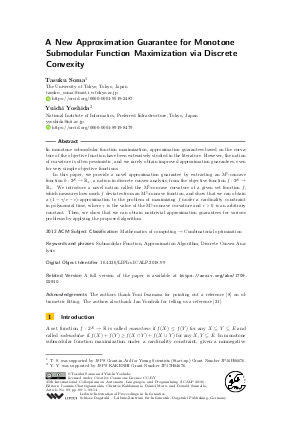Document# A New Approximation Guarantee for Monotone Submodular Function Maximization via Discrete Convexity

### Authors Tasuku Soma, Yuichi Yoshida## File

LIPIcs.ICALP.2018.99.pdf
• Filesize: 495 kB
• 14 pages

## Cite As

Tasuku Soma and Yuichi Yoshida. A New Approximation Guarantee for Monotone Submodular Function Maximization via Discrete Convexity. In 45th International Colloquium on Automata, Languages, and Programming (ICALP 2018). Leibniz International Proceedings in Informatics (LIPIcs), Volume 107, pp. 99:1-99:14, Schloss Dagstuhl - Leibniz-Zentrum für Informatik (2018)
https://doi.org/10.4230/LIPIcs.ICALP.2018.99

## Abstract

In monotone submodular function maximization, approximation guarantees based on the curvature of the objective function have been extensively studied in the literature. However, the notion of curvature is often pessimistic, and we rarely obtain improved approximation guarantees, even for very simple objective functions. In this paper, we provide a novel approximation guarantee by extracting an M^{natural}-concave function h:2^E -> R_+, a notion in discrete convex analysis, from the objective function f:2^E -> R_+. We introduce a novel notion called the M^{natural}-concave curvature of a given set function f, which measures how much f deviates from an M^{natural}-concave function, and show that we can obtain a (1-gamma/e-epsilon)-approximation to the problem of maximizing f under a cardinality constraint in polynomial time, where gamma is the value of the M^{natural}-concave curvature and epsilon > 0 is an arbitrary constant. Then, we show that we can obtain nontrivial approximation guarantees for various problems by applying the proposed algorithm.

## Subject Classification

##### ACM Subject Classification
• Mathematics of computing → Combinatorial optimization
##### Keywords
• Submodular Function
• Approximation Algorithm
• Discrete Convex Analysis

## Metrics

• Access Statistics
• Total Accesses (updated on a weekly basis)
0

## References

1. Eric Balkanski, Aviad Rubinstein, and Yaron Singer. The power of optimization from samples. In Advances in Neural Information Processing Systems (NIPS), pages 4017-4025, 2016.2. Gruia Calinescu, Chandra Chekuri, Martin Pál, and Jan Vondrák. Maximizing a monotone submodular function subject to a matroid constraint. SIAM Journal on Computing, 40(6):1740-1766, 2011.3. Vaggos Chatziafratis, Tim Roughgarden, and Jan Vondrak. Stability and recovery for independence systems. In Proceedings of ALGO, 2017.4. Chandra Chekuri, Jan Vondrák, and Rico Zenklusen. Dependent randomized rounding via exchange properties of combinatorial structures. In Proceedings of IEEE 51st Annual Symposium on Foundations of Computer Science (FOCS), pages 575-584, 2010.5. Chandra Chekuri, Jan Vondrák, and Rico Zenklusen. Submodular function maximization via the multilinear relaxation and contention resolution schemes. SIAM Journal on Computing, 43(6):1831-1879, 2014.6. Michele Conforti and Gérard Cornuéjols. Submodular set functions, matroids and the greedy algorithm: Tight worst-case bounds and some generalizations of the Rado-Edmonds theorem. Discrete Applied Mathematics, 7(3):251-274, 1984.7. Shahar Dobzinski, Noam Nisan, and Michael Schapira. Approximation algorithms for combinatorial auctions with complement-free bidders. Mathematics of Operations Research, 35(1):1-13, 2010.8. M. Farach, S. Kannan, and Tandy Warnow. A robust model for finding optimal evolutionary trees. Algorithmica, 13:155-179, 1995. URL: http://dx.doi.org/10.1007/BF01188585.
9. Hiroshi Hirai and Kazuo Murota. M-convex functions and tree metrics. Japan Journal of Industrial and Applied Mathematics, 21(3):391-403, 2004.10. Rishabh Iyer and Jeff Bilmes. Submodular optimization with submodular cover and submodular knapsack constraints. In Advances in Neural Information Processing Systems (NIPS), pages 2436-2444, 2013.11. David Kempe, Jon Kleinberg, and Éva Tardos. Maximizing the spread of influence through a social network. In Proceedings of the 9th ACM SIGKDD International Conference on Knowledge Discovery and Data Mining (KDD), pages 137-146, 2003.12. Andreas Krause and Carlos Guestrin. Submodularity and its applications in optimized information gathering. ACM Transactions on Intelligent Systems and Technology, 2(4):32-20, 2011.13. Andreas Krause, Ajit Paul Singh, and Carlos Guestrin. Near-optimal sensor placements in gaussian processes: Theory, efficient algorithms and empirical studies. Journal of Machine Learning Research, pages 235-284, 2008.14. Benny Lehmann, Daniel J Lehmann, and Noam Nisan. Combinatorial auctions with decreasing marginal utilities. Games and Economic Behavior, 55:270-296, 2006.15. Hui Lin and Jeff Bilmes. Learning mixtures of submodular shells with application to document summarization. In Uncertainty in Artificial Intelligence (UAI), 2012.16. Kazuo Murota. Discrete Convex Analysis. Society for Industrial and Applied Mathematics, 2003.17. Kazuo Murota. Discrete convex analysis: A tool for economics and game theory. Journal of Mechanism and Institution Design, 1(1):151-273, 2016.18. Kazuo Murota and Akiyoshi Shioura. M-convex function on generalized polymatroid. Mathematics of Operations Research, 24(1):95-105, 1999.19. Kazuo Murota and Akiyoshi Shioura. Extension of m-convexity and L-convexity to polyhedral convex functions. Advances in Applied Mathematics, 25(4):352-427, nov 2000.20. G. L. Nemhauser, L. A. Wolsey, and M. L. Fisher. An analysis of approximations for maximizing submodular set functions - I. Mathematical Programming, 14(1):265-294, 1978.21. Tim Roughgarden, Inbal Talgam-Cohen, and Jan Vondrák. When are welfare guarantees robust? In Approximation, Randomization, and Combinatorial Optimization. Algorithms and Techniques (APPROX/RANDOM)., 2017.22. Akiyoshi Shioura. On the pipage rounding algorithm for submodular function maximization - a view from discrete convex analysis - . Discrete Mathematics, Algorithms and Applications, 1(1):1-23, 2009.23. Akiyoshi Shioura. Polynomial-time approximation schemes for maximizing gross substitutes utility under budget constraints. Mathematics of Operations Research, 40(1):192-225, 2015.24. Maxim Sviridenko, Jan Vondrák, and Justin Ward. Optimal approximation for submodular and supermodular optimization with bounded curvature. In Proceedings of the 26th Annual ACM-SIAM Symposium on Discrete Algorithms (SODA), pages 1134-1148, 2015.25. Jan Vondrák. Submodularity and curvature: the optimal algorithm. RIMS kokyuroku Bessatsu, 23:253-266, 2010.26. Yuichi Yoshida. Maximizing a monotone submodular function with a bounded curvature under a knapsack constraint. arXiv preprint, 2016.X

Feedback for Dagstuhl Publishing